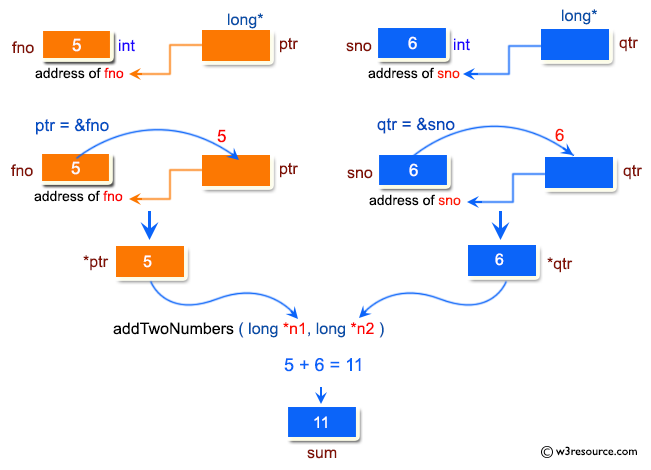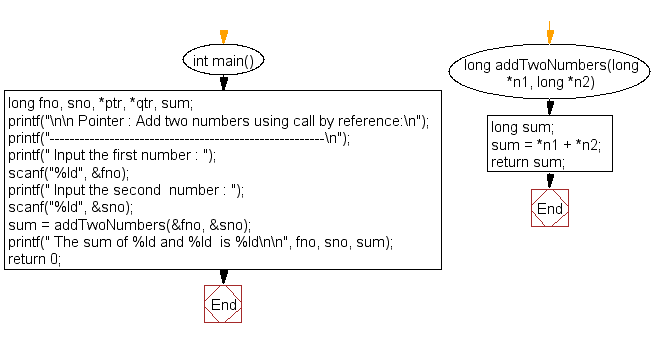﻿ C exercises: Add two numbers using call by reference - w3resourceC Exercises: Add two numbers using call by reference

C Pointer : Exercise-5 with Solution

Write a program in C to add numbers using call by reference.

Pictorial Presentation:Sample Solution:

C Code:

``````#include <stdio.h>

int main()
{
long fno, sno, *ptr, *qtr, sum;

printf("\n\n Pointer : Add two numbers using call by reference:\n");
printf("-------------------------------------------------------\n");

printf(" Input the first number : ");
scanf("%ld", &fno);
printf(" Input the second  number : ");
scanf("%ld", &sno);
printf(" The sum of %ld and %ld  is %ld\n\n", fno, sno, sum);
return 0;
}
{
long sum;
sum = *n1 + *n2;
return sum;
}
```
```

Sample Output:

``` Pointer : Add two numbers using call by reference:
-------------------------------------------------------
Input the first number : 5
Input the second  number : 6
The sum of 5 and 6  is 11
```

Flowchart:C Programming Code Editor:

Have another way to solve this solution? Contribute your code (and comments) through Disqus.

What is the difficulty level of this exercise?

﻿

New Content: Composer: Dependency manager for PHP, R Programming

bedava porno